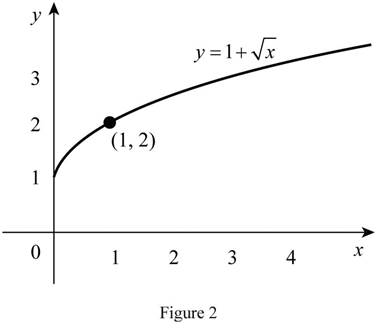# The graph of the function y = 2 sin x by using the graph of y = sin x and explain how the graph y = 2 sin x is related to y = sin x .### Single Variable Calculus: Concepts...

4th Edition
James Stewart
Publisher: Cengage Learning
ISBN: 9781337687805### Single Variable Calculus: Concepts...

4th Edition
James Stewart
Publisher: Cengage Learning
ISBN: 9781337687805

#### Solutions

Chapter 1.3, Problem 8E

(a)

To determine

## To sketch: The graph of the function y=2sinx by using the graph of y=sinx and explain how the graph y=2sinx is related to y=sinx .

Expert Solution

### Explanation of Solution

Since 2 multiplied by sinx , stretch the graph y=sinx vertically by a factor of 2. Thus, the graph of y=2sinx is shown below in Figure 1.From Figure 1, it is observed that the sine curve is vertically stretched by a factor of 2.

(b)

To determine

Expert Solution

### Explanation of Solution

Since 1 added with x , shift the graph of y=x by 1 unit upward. Thus, the graph of y=1+x is shown below in Figure 2.From Figure 2, it is observed that the graph y=x is shifted upward by 1 unit. Also, observe that the point (1, 2) is satisfying the equation y=1+x .

### Have a homework question?

Subscribe to bartleby learn! Ask subject matter experts 30 homework questions each month. Plus, you’ll have access to millions of step-by-step textbook answers!